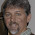## Sunday, April 08, 2007

### Get Variable Type

To know the data type of the variable:

Function Get_Variable_Type(myVar)

' ---------------------------------------------------------------
' Written By Shanmuga Sundara Raman for http://vbadud.blogspot.com
' ---------------------------------------------------------------

If VarType(myVar) = vbNull Then
MsgBox "Null (no valid data) "
ElseIf VarType(myVar) = vbInteger Then
MsgBox "Integer "
ElseIf VarType(myVar) = vbLong Then
MsgBox "Long integer "
ElseIf VarType(myVar) = vbSingle Then
MsgBox "Single-precision floating-point number "
ElseIf VarType(myVar) = vbDouble Then
MsgBox "Double-precision floating-point number "
ElseIf VarType(myVar) = vbCurrency Then
MsgBox "Currency value "
ElseIf VarType(myVar) = vbDate Then
MsgBox "Date value "
ElseIf VarType(myVar) = vbString Then
MsgBox "String "
ElseIf VarType(myVar) = vbObject Then
MsgBox "Object "
ElseIf VarType(myVar) = vbError Then
MsgBox "Error value "
ElseIf VarType(myVar) = vbBoolean Then
MsgBox "Boolean value "
ElseIf VarType(myVar) = vbVariant Then
MsgBox "Variant (used only with arrays of variants) "
ElseIf VarType(myVar) = vbDataObject Then
MsgBox "A data access object "
ElseIf VarType(myVar) = vbDecimal Then
MsgBox "Decimal value "
ElseIf VarType(myVar) = vbByte Then
MsgBox "Byte value "
ElseIf VarType(myVar) = vbUserDefinedType Then
MsgBox "Variants that contain user-defined types "
ElseIf VarType(myVar) = vbArray Then
MsgBox "Array "
Else
MsgBox VarType(myVar)
End If

' Excel VBA, Visual Basic, Get Variable Type, VarType

End Function

1.Anonymous6:19 PM

That's a very nice pice of code, but if you would like a much simpler wat to acheive much the same thing, then try this:

MsgBox TypeName(VARIABLE_GOES_HERE)

And seriously, that's all you need!

2.Anonymous5:37 AM

Hi,

Not one of those keywords are recognised : vartype or typename...

Is it VBA with excel ?

1.Anonymous9:16 AM

You must be a Newbie, because varType is not a keyword. It's just a proceedure's local variable.
If you want Shasur's Proceedure's to work for you jus add it to a module and then add a UDF one and call it from there.

Ex.

Sub FindTypeName()
myVar = True
Get_Variable_Type (myVar)
End Sub

Hope it helps.
ATH

2.Anonymous10:35 AM

What are you talking about? VarType is in no way a procedure's local variable. It is a built-in VBA function. Even if it were not a reserved keyword (which it is), it wouldn't be a variable at all, local or otherwise.

3.Anonymous6:58 PM

Yes, this is on VBA with excel, and it works fine with mine. (Though I am using Excel 2007, so not sure if it was supported on older versions) It may also be that you have some libraries disabled. But if you check out the TypeName variable in microsoft help, it should point you to which library to enable. Here's a link to the usage if the TypeName function http://office.microsoft.com/en-us/access-help/typename-function-HA001228928.aspx

1.Not "in VBA with Excel", but in VBA, period. It works as well in Word, Outlook, and outside Office, since VBA is not by design limited to Office.

4.Anonymous7:04 PM

P.S. In case you were looking for the .NET version of the same thing then use TypeOf instead of TypeName. ;)

5.TypeName(Variable) also works with 2010. This is a great function if you are looking to discern a variant input to a user defined function.

We may have different code for handling an Input Range versus a String supplied directly.

Function UDF(Variable as Variant) as String
UDF = TypeName(Variable)
End Function

6.I'm using MS Office 2003 and Redge's UDF function works great.

7.There are several conflicting comments here.
Using Excel 2010, VARTYPE and TYPENAME are indeed keywords.

TYPENAME works just as advertised: TypeName(Variable)

VARTYPE works just as advertised: VarType(Variable)

However, TYPEOF is only useful for object variables.

Cheers,

Mitch

1.You mean TYPENAME, not TYPEOF.

You say it's only useful for object variables, this deserves an explanation.

To test the type of "base type" variable V such as Integer, you may write (all the following Debug.Print will print "True")

V = 1
Debug.Print VarType(V) = vbInteger
Debug.Print TypeName(V) = "Integer"

The former is simpler, as it only involves a VBA integer constant (vbInteger).

With an object such as Range (in Excel)

Set V = Range("A1")
Debug.Print VarType(V) = vbEmpty
Debug.Print TypeName(V) = "Range"

Hence, only the latter gives a useful information.Download Windows Live Toolbar and personalize your Web experience! Add custom buttons to get the information you care about most.